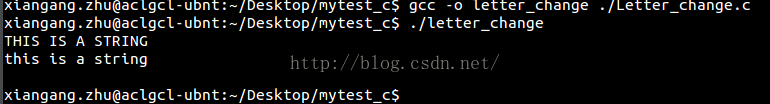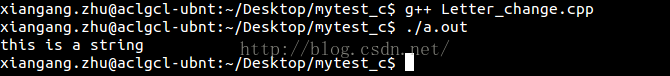# C / C + + library function (tower / tower) realizes the conversion of letter case

C/C++ library function (tolower/toupper) implements case conversion of letters

This article will introduce the library function to implement the case conversion of letters, commonly used in the type. H (c ++ is cctype) library file under the definition of function methods. First let’s look at the prototype implementation of the tolower/toupper function under C:

``````int tolower(int c)
{
if ((c >= 'A') && (c <= 'Z'))
return c + ('a' - 'A');
return c;
}

int toupper(int c)
{
if ((c >= 'a') && (c <= 'z'))
return c + ('A' - 'a');
return c;
}``````

And I’m going to do that with two little demos.

C implementation:

``````#include<string.h>   //strlen
#include<stdio.h>    //printf
#include<ctype.h>    //tolower
int main()
{
int i;
char string[] = "THIS IS A STRING";
printf("%s\n", string);
for (i = 0; i < strlen(string); i++)
{
string[i] = tolower(string[i]);
}
printf("%s\n", string);
printf("\n");
}``````

Save as xxX. c file, execute: GCC-O XXX xxX. c generate execute file XXX. Operation:./ XXXabove is the implementation of C. Similarly, the implementation under C++ is as follows:

``````#include <iostream>
#include <string>
#include <cctype>
using namespace std;
int main()
{
string str= "THIS IS A STRING";
for (int i=0; i <str.size(); i++)
str[i] = tolower(str[i]);
cout<<str<<endl;
return 0;
}``````

Save as xxx. CPP, execute g++ xxx. CPP to generate the execution file a.ut, execute a.ut, and the effect is as follows:The demo above
implements the upper case tolower case conversion, again, the lower case toupper case conversion is the same, just replace tolower with toupper.